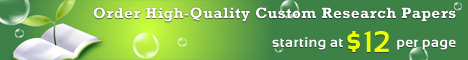## Free Research Paper on Mathematics

Mathematics
is the language of science that provides tools necessary for deeper analysis of scientific concepts and applications.

Many connections can be found between math and science. Elements such as graphing, geometry, and basic algebra are a few concepts used to explain scientific ideas. In fact, several scientific discoveries and theories are based on mathematical equations alone. Many scientists have used mathematics as the basis of their discoveries. People like Louis Pastuer, Johannes Kepler, and Neils Bohr have titles of Biologists, Physicists, and Astronomers. In all actuality their work has proven them to be mathematicians as well.
Many aspects of science are based on geometrical concepts that are used to express the forms and structures of such phenomena (Tracy, 200). Astronomy encompasses elements such as symmetrical formations and elliptical orbits that can only be expressed through a geometrical framework (Tracy, 195). Past Astronomers based much of their findings on geometry alone.

We can write a Custom Research Paper on Mathematics for you!

Johannes Kepler was one of the first great developers in the history of astronomy. A German sent to Prague, Bohemia (now the Czech Republic), became the assistant to a man by the name of Tycho Brahe (Gingerich, 116). The two did not get along well. It has been said that Brahe actually did not trust Kepler and was afraid that his bright, young assistant might overshadow him (Gingerich, 116). To keep Kepler busy Brahe gave him the task of understanding the orbit of the planet Mars, which was particularly troublesome. It is believed that part of the reason Brahe gave the Mars problem to Kepler was because he hoped it would occupy Kepler while Brahe worked on his theory of the Solar System (Gingerich, 118). Ironically, the Mars data allowed Kepler to formulate the correct laws of planetary motion.

Kepler firmly believed in the Copernican system, which includes seven axioms that Copernicus used to formulate his conclusions. The most prevalent of the seven axioms was the fact that he believed the sun was at the center of the universe (Gingerich, 120). This idea confused Kepler thus leading him to research further. While doing research on Mars, Kepler concluded that the orbit of the planet was not circular, rather it was elliptical (http://www.csep10.phys.utk.edu/astr161/lect/kepler.html).

For an ellipse there are two points called foci (focus for singular) such that the sum of the distances to the foci from any point on the ellipse is a constant (Gingerich, 121). The diagram below shows the foci marked with an “x”. This gives the equation of a + b = constant. This defines the ellipse in terms of distances a and b. (http://www.csep10.phys.utk.edu/astr161/lect/kepler.html).

From Kepler?s discovery of elliptical orbits he was able to formulate his Three Laws of Planetary Motion. The First Law uses geometrical concepts to explain why the orbits of the planets are ellipses, with the sun at one focus of the ellipse (Gingerich, 121). Kepler’s Second Law demonstrates several aspects of geometry. First, he used elliptical shapes to represent the orbit of the planets. Next, Kepler gives an example of measuring the effect of a shadow by stating that the Sun sweeps out equal areas in equal times as the planet travels around the ellipse (Gingerich, 121). Below are Johannes Kepler’s First and Second Laws of Planetary Motion (http://www.csep10.phys.utk.edu/astr161/lect/kepler.html).

Kepler?s Third Law of Planetary Motion was developed using algebraic equations, rather than geometrical shapes and patterns. This law shows how the ratio of the squares of the revolutionary periods for two planets is equal to the ratio of the cubes of their semi-major axes (Gingerich, 122). In this equation, P represents the period and R represents the length of its semi-major axes. The subscripts “1” and “2” distinguish quantities for planet 1 and 2 respectively. Thus, Kepler’s Third Law of Planetary Motion is (http://www.csep10.phys.utk.edu/astr161/lect/kepler.html):

Astronomy and the work of Johannes Kepler makes clear that mathematical concepts are integral to science. In his work, we saw that geometry and algebra are used to explain scientific concepts. Other aspects of mathematics, for example, graphing are also used in science. Understanding graphs is essential for scientists because graphs are a visual way of representing information obtained through study and observation. Graphs can often reveal trends, tendencies or patterns in data that are not evident by examining a long list of handwritten data (Charlesworth, 88). Many areas within the sciences use graphs for these reasons. In Physics, scientists and students use graphs to plot data such as mass versus volume and time versus temperature. Astronomers often plot data such as the period of time the sun casts a shadow on a given object. Graphs are simply another form of supplying data and results.Free research papers, free research paper samples and free example research projects on Mathematics topics are plagiarized. EffectivePapers.com is professional research paper writing service which is committed to write top-quality custom research papers, term papers, essays, thesis papers and dissertations. All custom research papers are written by qualified Master’s and PhD writers. Just order a custom written research paper on at our website and we will write your research paper at affordable prices. We are available 24/7 to help students with writing research papers for high school, college and university.

## 2 thoughts on “Free Research Paper on Mathematics”

1.Daniel Tsado

i like it

2.JESYWIN R. PENIDO

My daughter is asking for help from for sample of research involving Mathematics of Investment I am not familiar with how to make it could you please send me a sample of it Thank you and more power. God Bless!